Tip: Other languages are Google-Translated. You can visit the English version of this link.orRegisteror

## Excel FORMULATEXT function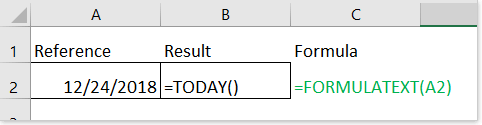Description

Syntax and Arguments

Usage and Examples

### Description

The FORMULATEXT function is used to display or return a formula as a text string from a given reference.

### Syntax and Arguments

Formula syntax

 FORMULATEXT(reference)

Arguments

 Reference: Required, a cell or a range of cells. If the reference is a range of cell, it will return the text string of formula applied in the first cell of this range.

Return Value

The FORMULATEXT function returns a formula as text string.

The result is #N/A error value if:
1) The reference cell does not contain a formula;
2) The formula in the referenced cell is longer than 8192 characters;
3) Worksheet is protected from displaying formula;
4) The external workbook which contains formula is closed.

The result is #VALUE! error value if:
1) Some invalid character typed in function.

### Usage and Examples

Here I take some simple examples to explain how to use the FORMULATEXT function in Excel.

Ex1: Basic use-display cell’s formula

Formula

=FORMULAR(A2)

A1 contains the formula =TODAY() and returns today’ s date,

B2 contains the formula =FORMULATEXT(A2) and returns the text string what displays in the formula bar from cell A2

Result: Display the formula applied in Cell A2 as a text string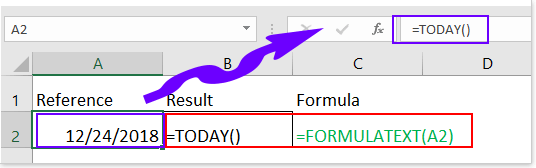Ex2: Count length of formula

Formula

=LEN((FORMULATEXT(A3)))

Explain:

LEN: count characters of a cell

LEN((FORMULATEXT(reference))): count the number of characters of formula from reference.

Result: Count the length of formula In Cell A3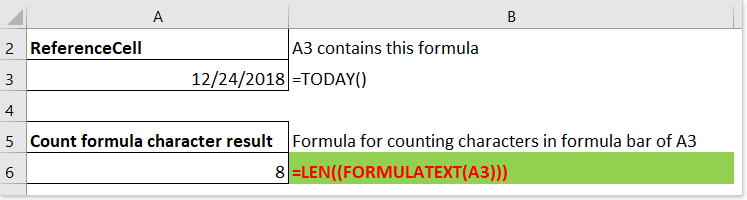Ex3: Display formula of another cell

Formula

=FORMULATEXT(INDIRECT(B5))

Explain:

INDIRECT(B5): display the value in Cell B5. in my example, the Cell B5 contains the value “B2”.

then

FORMULARTEXR(INDIRECT(B5)) equals to FORMULATEXT(B2)

Result: Display the formula applied in Cell B2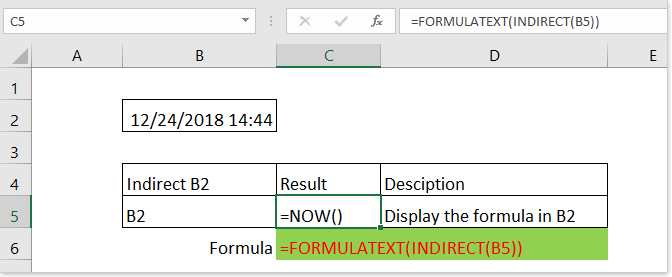Ex4: Display formula or a message

If the reference not contain a formula, the FORMULATEXT function will returns #N/A error value as below screenshot shown. To avoide the error value appearing, you can combine the ISFORMULA function and the FORMULATEXT function to display formula or a message based on the reference.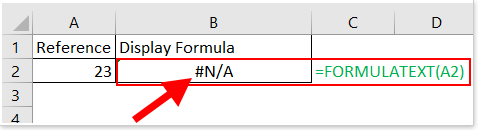Formula

=IF(ISFORMULA(A2),FORMULATEXT(A2),"not a formula")

Explain

If Cell A2 contains a formula, then execute the FORMULATEXT function; if not, display the text string “not a formula”.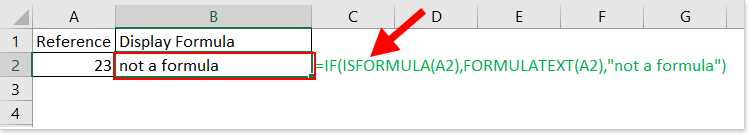Note:This FORMULATEXT functions is a newly added since Excel 2013

### Excel Productivity Tools

Would you like to complete your daily work quickly and perfectly? Kutools for Excel brings 300 powerful advanced features (Combine workbooks, sum by color, split cell contents, convert date, and so on...) and save 70% time.

• Designed for 1500 work scenarios, helps you solve 80% Excel problems.
• Reduce thousands of keyboard and mouse clicks every day, relieve your tired eyes and hands.
• Become an Excel expert in 3 minutes. No longer need to remember any painful formulas and VBA codes.
• 60-day unlimited free trial. 60-day money back guarantee. Free upgrade and support for 2 years.

#### Brings Efficient Tabs to Office (include Excel), Just Like Chrome, Firefox, And New IE

• Office Tab Increases your productivity by 50% when viewing and editing multiple documents.
• Reduce hundreds of mouse clicks for you every day, say goodbye to mouse hand.
• One second to switch between dozens of open documents!Say something here...
symbols left.